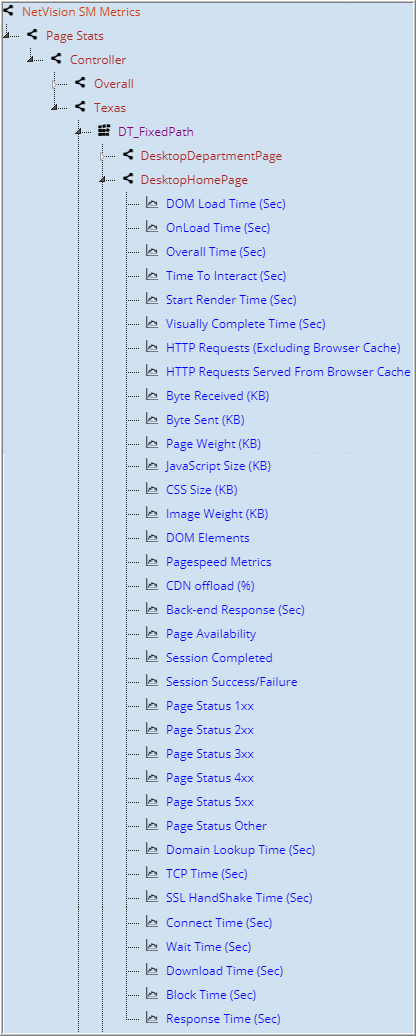# NetVision SM Metrics

NetVision SM Metrics is a collection of NetVision SM graphs. These graphs can be seen on the right pane of the web dashboard window under Advanced options.• DOM Load Time: Average DOM content load time in seconds, where DOM content is considered loaded when the engine has completed parsing of the main document and the DOMContentLoad event is fired.
• OnLoad Time: Average OnLoad time in seconds where OnLoad time is the time until all the resources referenced by the page up until onload has loaded (images, script) is thrown and finished executing.
• Overall Time: Average page load time in seconds where page load time is the time the webpage actually took to load all the resources of the web page.
• Time to Interact: Average time taken by a web page to get interactive. Time to interact is defined as the point at which a page displays its primary interactive content.
• Start Render Time: Average start render time in seconds where start render in time when something first displays on the user’s screen.
• Visually Complete Time: Average visually completed time in seconds where visually completed time is where user’s screen is fully loaded.
• HTTP Requests (Excluding Browser Cache): Average number of HTTP requests excluding browser cache per page.
• HTTP Requests Served from Browser Cache: Average number of resource served by browser cache per page.
• Byte Received: Average total number of bytes received for a page.
• Byte Sent: Average total number of bytes sent for a page.
• Page Weight: Average page weight (Page weight= sum of JavaScript size, CSS size, and image weight)
• JavaScript Size: This denoted the average JavaScript size.
• CSS Size: This denotes the average CSS size.
• Image Weight: This denotes the average of Image weight.
• DOM Elements: This denotes the average of total number of DOM elements in page.
• Pagespeed Metrics: This denotes the Google ranking of the page.
• CDN Offload: This denotes the average percentage of requests served by content delivery network (CDN) such as Akamai, Strangeloop, and Instart
• Backend Response: This denotes the average page load time in seconds where page load time is the time the web page actually took to load all the resources of the page.
• Page Availability: This denotes the average of page availability.
• Session Completed: This denotes the total number of Sessions completed.
• Session Success/Failure: This denotes the total number of sessions with success/failure status.
• Page Status 1xx: This graphs denotes the page with informational responses.
• Page Status 2xx: This graph denotes the pages with success status.
• Page Status 3xx: This graph denotes the pages having redirection on network.
• Page Status 4xx: This graph denotes the pages with client error.
• Page Status 5xx: This graph denotes the pages with server error.
• Page Status Other: This denotes the pages with various other status, such as MiscErr, TimeOut, etc.
• Domain Lookup Time (Sec): This denotes the domain lookup time in seconds.
• TCP time (Sec): This denotes the TCP time in seconds.
• SSL HandShake Time (Sec): This denotes the SSL Handshake time in seconds.
• Connect Time (Sec): This denotes the connection time in seconds.
• Wait Time (Sec): This denotes the wait time in seconds.